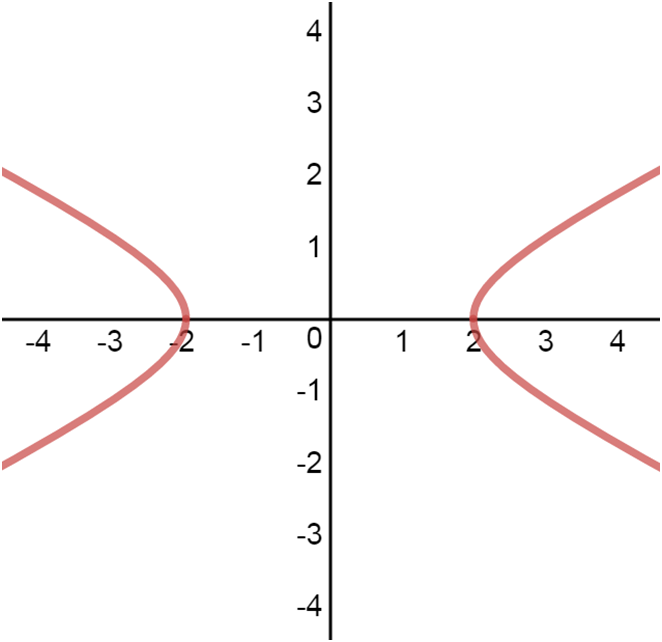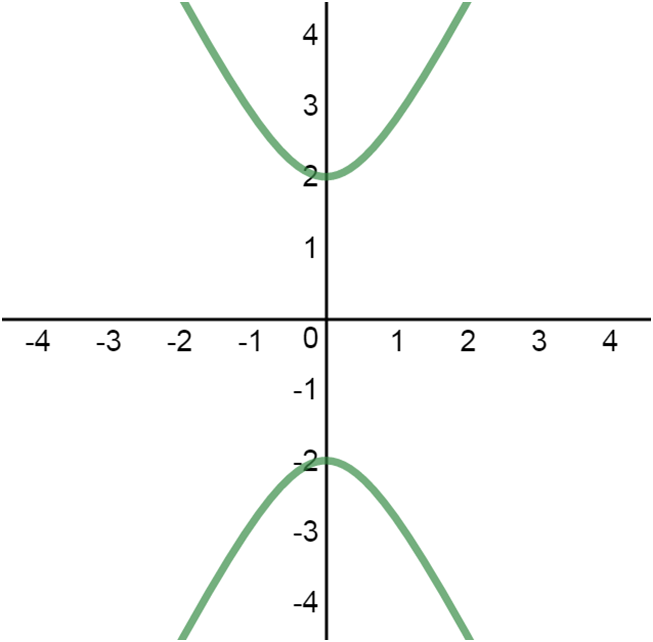# Conics - Hyperbola##### Introduction
###### Lessons
1. Hyperbola:
horizontal hyperbolavertical hyperbola• Graph looks sort of like two mirrored parabolas, with the two "halves" being called "branches".
• "Vertices" are defined similarly to the way of a "vertex" is defined for a parabola.
• Just as the focus for a parabola, the two foci for a hyperbola are inside each branch.
• The line connecting the two vertices is called the "transverse axis".
##### Examples
###### Lessons
1. properties of a hyperbola
$-(\frac{x-6}{4})^2 + (\frac{y+5}{3})^2=1$
1. Identify the type of conic section.
2. State the "center".
3. Set up the guidelines for the conic graph.
4. Find the "vertices".
5. Locate the "foci".
6. Find the "eccentricity".
7. Find the equations of the "asymptotes".
8. Find the lengths of transverse axis and conjugate axis.
###### Free to Join!
StudyPug is a learning help platform covering math and science from grade 4 all the way to second year university. Our video tutorials, unlimited practice problems, and step-by-step explanations provide you or your child with all the help you need to master concepts. On top of that, it's fun - with achievements, customizable avatars, and awards to keep you motivated.
• #### Easily See Your ProgressWe track the progress you've made on a topic so you know what you've done. From the course view you can easily see what topics have what and the progress you've made on them. Fill the rings to completely master that section or mouse over the icon to see more details.
• #### Make Use of Our Learning Aids###### Practice Accuracy

See how well your practice sessions are going over time.

Stay on track with our daily recommendations.

• #### Earn Achievements as You LearnMake the most of your time as you use StudyPug to help you achieve your goals. Earn fun little badges the more you watch, practice, and use our service.
• #### Create and Customize Your AvatarPlay with our fun little avatar builder to create and customize your own avatar on StudyPug. Choose your face, eye colour, hair colour and style, and background. Unlock more options the more you use StudyPug.
###### Topic Notes
hyperbola: the difference of the distances from any point on a hyperbola to each focus is constant and equal to the transverse axis $2a$.
ellipse: the sum of the distances from any point on an ellipse to each focus is constant and equal to the major axis $2a$.

$c= \sqrt{a^2 - b^2}$ $c$: distance from the center to a focus

$e= \frac{c}{a}$ $e$: eccentricity; the larger the value of $e$, the straighter the hyperbola